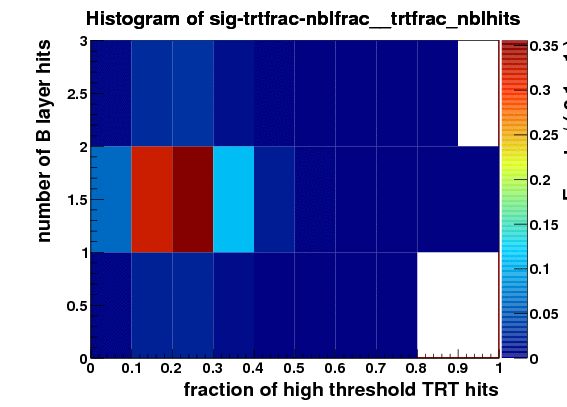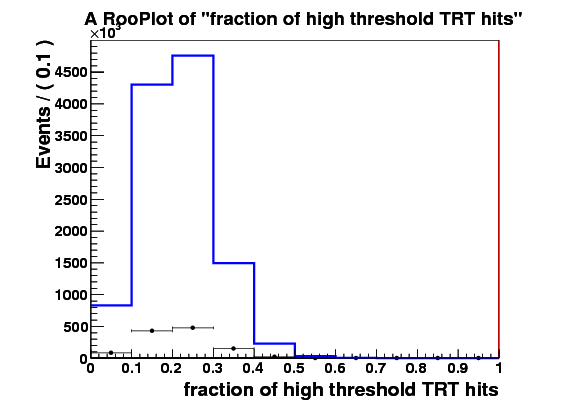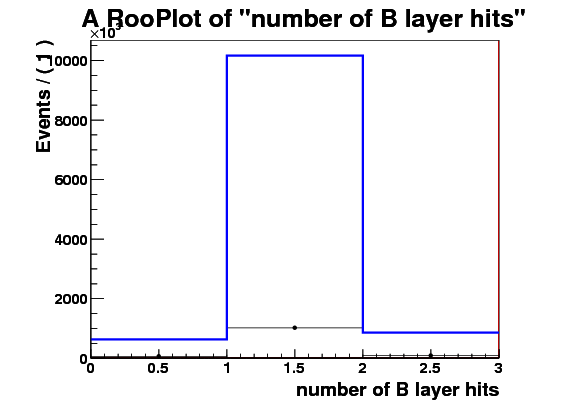# RooHistPdf normalization

Hi,
I am trying to create a 2d histogram pdf using RooFit. It seems that the normalization gets messed up.

I load a tree with about 1m entries from a file. Tree has 2 observables trtfrac and nblhits.
I make RooDataSet out of this and then make a RooDataHist out of this, and finally I make RooHistPdf. The trtfrac variable range is from 0.0 to 1.0 in 10 bins. and nblhits goes from 0 to 3 in 3 bins. Then I try to plot the projection of the pdf on both observables together with the dataset that the pdf was built from. I expect the two to lie exactly on top of one another. But - I get about factor of 10 difference - the pdf is data x 10. Also when I ask the pdf to give me a 2d histogram and I integrate that I get 0.1 instead of 1. These two factors are linked to the bin size in the trtfrac. So when I bin it twice as fine I get factor of 20 discrepancy in data plotted with the pdf and the pdf integral becomes 0.05.

I attach the relevant bit of code and 3 plots

RooRealVar trtfrac(“trtfrac”,“fraction of high threshold TRT hits”,0.0,1.0);
trtfrac.setBinning(RooBinning(10,0.0,1.0));

RooRealVar nblhits(“nblhits”,“number of B layer hits”,0.0,3.0);
RooBinning nblhits_bin(3,0.0,3.0);
nblhits.setBinning(nblhits_bin);

cerr<<“open file get tree: “<<sig_file_name<<” “<<sig_tree_name<<”\n”;
TFile* f_sig=new TFile(sig_file_name.c_str());
TTree* t_sig_trtfrac_nblhits=(TTree*)f_sig->Get(sig_tree_name.c_str());
//“t_trtfrac_nblhits_minimal_Et2p5GeV_fake”);

cerr<<“sig file tree: “<<f_sig<<” “<<t_sig_trtfrac_nblhits<<”\n”;

RooDataSet sig_data(“sig_data”,“signal training dataset”,
t_sig_trtfrac_nblhits,RooArgSet(trtfrac,nblhits));
cerr<<“number of entries in the dataset: “<<sig_data.numEntries()<<”\n”;

RooDataHist hist_sig_trtfrac_nblhits(“hist_sig_trtfrac_nblhits”,“Number of B Layer hits VS TR fraction”, RooArgList(trtfrac,nblhits),sig_data);

cerr<<“num entries: “<<t_sig_trtfrac_nblhits->GetEntries()<<”\n”;

std::cerr<<“making the pdf!\n”;

RooHistPdf sig_PDF(“sig_PDF”,“signal PDF”,RooArgSet(trtfrac,nblhits),hist_sig_trtfrac_nblhits);

std::cerr<<“done\n”;
TCanvas* c_sig_frame=new TCanvas(“c_sig_frame”,“c_sig_frame”,800,600);
c_sig_frame->cd();

TH2F* sig_PDF_histo=(TH2F*)sig_PDF.createHistogram(“sig-trtfrac-nblfrac”,trtfrac,YVar(nblhits));
sig_PDF_histo->Draw(“COLZ”);
c_sig_frame->Print(“sig_PDF.eps”,“eps”);

RooPlot* sig_frame_trtfrac=trtfrac.frame();
RooPlot* sig_frame_nblhits=nblhits.frame();

//hist_sig_trtfrac_nblhits.plotOn(sig_frame_trtfrac);
sig_data.plotOn(sig_frame_trtfrac);
sig_PDF.plotOn(sig_frame_trtfrac);
sig_frame_trtfrac->Draw();
c_sig_frame->Print(“sig_PDF_trtfrac.eps”,“eps”);
//hist_sig_trtfrac_nblhits.plotOn(sig_frame_nblhits);
sig_data.plotOn(sig_frame_nblhits,Binning(nblhits_bin));
sig_PDF.plotOn(sig_frame_nblhits);
sig_frame_nblhits->Draw();
c_sig_frame->Print(“sig_PDF_nblhits.eps”,“eps”);
cerr<<“Integral of the 2d sig PDF histo: “<<
sig_PDF_histo->Integral(“width”)<<”\n”;

any help greatly appreciated!!!

also:
root version:
ROOT 5.22/00h (branches/v5-22-00-patches@32279, Feb 16 2010, 09:13:00 on linux)
RooFit v2.95

thanks!
WojtekHi,

(Sorry for the late reply. I was on vacation, which was involuntarily extended by an ash cloud)

Can you confirm that the problem is also present in the most recent ROOT version (5.27)?

Wouter